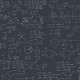Probability

# Sources For Probability

Let me start by telling why I wrote this article. While preparing for ISI, CMI I faced problems when faced with probability concepts like Independent events,Dependent events,Bayes Theorem and other related topics. Over time I learnt these topics and gained enough knowledge to do tough problems in these topics. I will be providing various sources so that you, my readers will be benefited.

• Discrete Probability by Hugh Gordon

This book was recommended by B.J.Venkatachala sir. It explains everything from basics and is a good read for beginners. The Random Variables chapter and parts thereof can be ignored. Link to the book is given below,

http://gen.lib.rus.ec/search.php?req=978-0387982274&lg_topic=libgen&open=0&view=simple&res=25&phrase=1&column=def

• A First Course In Probability by Sheldon Ross

• Probability through Problems

An advanced book. More abstract, but has some very good problems. Do try the problems in Chapter 7. Link,

http://gen.lib.rus.ec/search.php?req=978-0387950631&open=0&res=25&view=simple&phrase=1&column=def

## 3 thoughts on “Sources For Probability”

1.mathematicalworldblog says:

Liked by 1 person

1.knwitorlearn says:

I haven’t personally read the book,I will ask around and let you know.

Liked by 1 person

2.Vicky Ricky says:

what book should i practice for ISI and CMI level probability problems .

Like